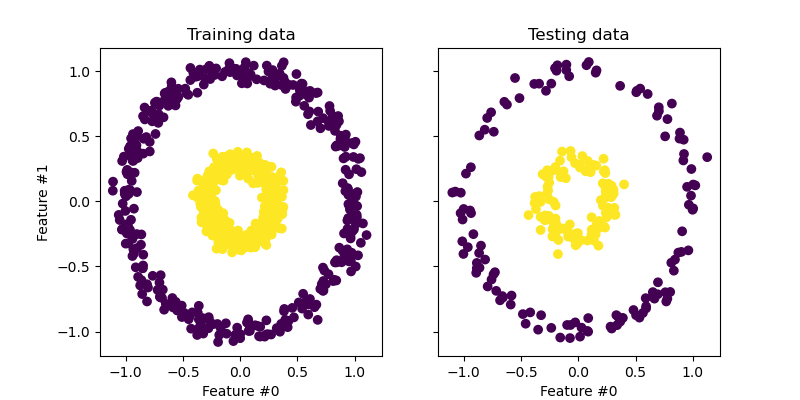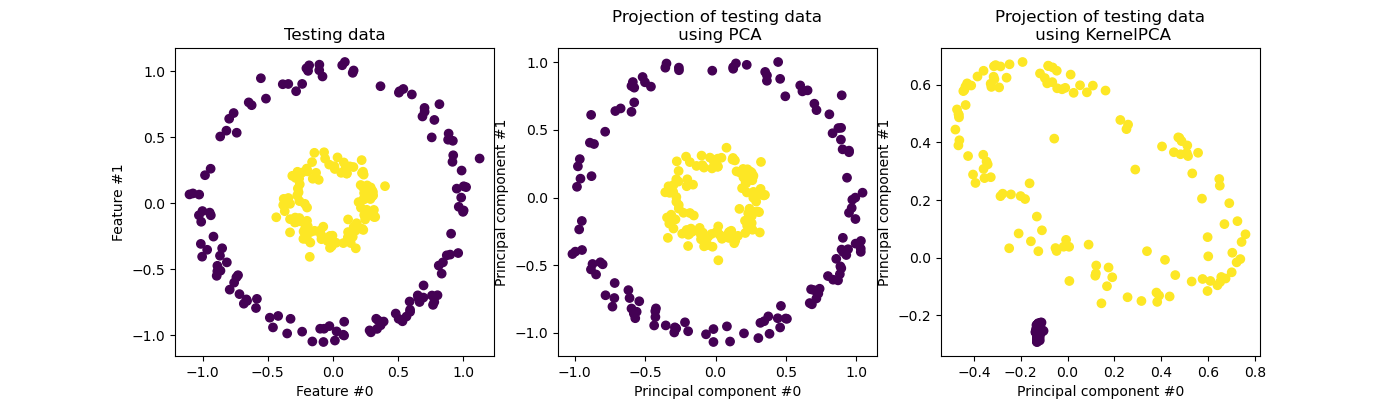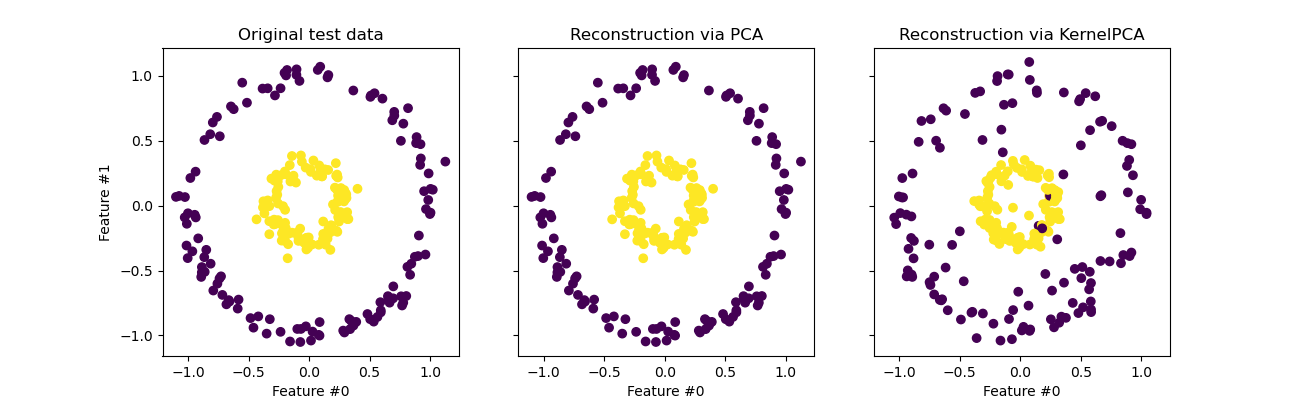# Kernel PCA¶

This example shows the difference between the Principal Components Analysis (PCA) and its kernalized version (KernelPCA).

On the one hand, we show that KernelPCA is able to find a projection of the data which linearly separates them while it is not the case with PCA.

Finally, we show that inverting this projection is an approximation with KernelPCA, while it is exact with PCA.

# Authors: Mathieu Blondel
#          Andreas Mueller
#          Guillaume Lemaitre


## Projecting data: PCA vs. KernelPCA¶

In this section, we show the advantages of using a kernel when projecting data using a Principal Component Analysis (PCA). We create a dataset made of two nested circles.

from sklearn.datasets import make_circles
from sklearn.model_selection import train_test_split

X, y = make_circles(n_samples=1_000, factor=0.3, noise=0.05, random_state=0)
X_train, X_test, y_train, y_test = train_test_split(X, y, stratify=y, random_state=0)


Let’s have a quick first look at the generated dataset.

import matplotlib.pyplot as plt

_, (train_ax, test_ax) = plt.subplots(ncols=2, sharex=True, sharey=True, figsize=(8, 4))

train_ax.scatter(X_train[:, 0], X_train[:, 1], c=y_train)
train_ax.set_ylabel("Feature #1")
train_ax.set_xlabel("Feature #0")
train_ax.set_title("Training data")

test_ax.scatter(X_test[:, 0], X_test[:, 1], c=y_test)
test_ax.set_xlabel("Feature #0")
_ = test_ax.set_title("Testing data")The samples from each class cannot be linearly separated: there is no straight line that can split the samples of the inner set from the outer set.

Now, we will use PCA with and without a kernel to see what is the effect of using such a kernel. The kernel used here is a radial basis function (RBF) kernel.

from sklearn.decomposition import PCA, KernelPCA

pca = PCA(n_components=2)
kernel_pca = KernelPCA(
n_components=None, kernel="rbf", gamma=10, fit_inverse_transform=True, alpha=0.1
)

X_test_pca = pca.fit(X_train).transform(X_test)
X_test_kernel_pca = kernel_pca.fit(X_train).transform(X_test)

fig, (orig_data_ax, pca_proj_ax, kernel_pca_proj_ax) = plt.subplots(
ncols=3, figsize=(14, 4)
)

orig_data_ax.scatter(X_test[:, 0], X_test[:, 1], c=y_test)
orig_data_ax.set_ylabel("Feature #1")
orig_data_ax.set_xlabel("Feature #0")
orig_data_ax.set_title("Testing data")

pca_proj_ax.scatter(X_test_pca[:, 0], X_test_pca[:, 1], c=y_test)
pca_proj_ax.set_ylabel("Principal component #1")
pca_proj_ax.set_xlabel("Principal component #0")
pca_proj_ax.set_title("Projection of testing data\n using PCA")

kernel_pca_proj_ax.scatter(X_test_kernel_pca[:, 0], X_test_kernel_pca[:, 1], c=y_test)
kernel_pca_proj_ax.set_ylabel("Principal component #1")
kernel_pca_proj_ax.set_xlabel("Principal component #0")
_ = kernel_pca_proj_ax.set_title("Projection of testing data\n using KernelPCA")We recall that PCA transforms the data linearly. Intuitively, it means that the coordinate system will be centered, rescaled on each component with respected to its variance and finally be rotated. The obtained data from this transformation is isotropic and can now be projected on its principal components.

Thus, looking at the projection made using PCA (i.e. the middle figure), we see that there is no change regarding the scaling; indeed the data being two concentric circles centered in zero, the original data is already isotropic. However, we can see that the data have been rotated. As a conclusion, we see that such a projection would not help if define a linear classifier to distinguish samples from both classes.

Using a kernel allows to make a non-linear projection. Here, by using an RBF kernel, we expect that the projection will unfold the dataset while keeping approximately preserving the relative distances of pairs of data points that are close to one another in the original space.

We observe such behaviour in the figure on the right: the samples of a given class are closer to each other than the samples from the opposite class, untangling both sample sets. Now, we can use a linear classifier to separate the samples from the two classes.

## Projecting into the original feature space¶

One particularity to have in mind when using KernelPCA is related to the reconstruction (i.e. the back projection in the original feature space). With PCA, the reconstruction will be exact if n_components is the same than the number of original features. This is the case in this example.

We can investigate if we get the original dataset when back projecting with KernelPCA.

X_reconstructed_pca = pca.inverse_transform(pca.transform(X_test))
X_reconstructed_kernel_pca = kernel_pca.inverse_transform(kernel_pca.transform(X_test))

fig, (orig_data_ax, pca_back_proj_ax, kernel_pca_back_proj_ax) = plt.subplots(
ncols=3, sharex=True, sharey=True, figsize=(13, 4)
)

orig_data_ax.scatter(X_test[:, 0], X_test[:, 1], c=y_test)
orig_data_ax.set_ylabel("Feature #1")
orig_data_ax.set_xlabel("Feature #0")
orig_data_ax.set_title("Original test data")

pca_back_proj_ax.scatter(X_reconstructed_pca[:, 0], X_reconstructed_pca[:, 1], c=y_test)
pca_back_proj_ax.set_xlabel("Feature #0")
pca_back_proj_ax.set_title("Reconstruction via PCA")

kernel_pca_back_proj_ax.scatter(
X_reconstructed_kernel_pca[:, 0], X_reconstructed_kernel_pca[:, 1], c=y_test
)
kernel_pca_back_proj_ax.set_xlabel("Feature #0")
_ = kernel_pca_back_proj_ax.set_title("Reconstruction via KernelPCA")While we see a perfect reconstruction with PCA we observe a different result for KernelPCA.

Indeed, inverse_transform cannot rely on an analytical back-projection and thus an exact reconstruction. Instead, a KernelRidge is internally trained to learn a mapping from the kernalized PCA basis to the original feature space. This method therefore comes with an approximation introducing small differences when back projecting in the original feature space.

To improve the reconstruction using inverse_transform, one can tune alpha in KernelPCA, the regularization term which controls the reliance on the training data during the training of the mapping.

Total running time of the script: ( 0 minutes 0.564 seconds)

Gallery generated by Sphinx-Gallery